# Pierpont variation

(diff) ← Older revision | Latest revision (diff) | Newer revision → (diff)

One of the numerical characteristics of a function of several variables which can be considered as a multi-dimensional analogue of the variation of a function of one variable. Let a function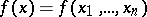,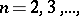be given on an-dimensional parallelopipedon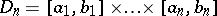and let,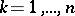, be a subdivision of the segment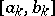into,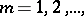equal segments by points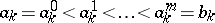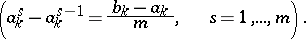These subdivisions generate a subdivision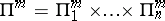of the parallelopipedonintoparallelopipeda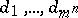with edges parallel to the coordinate axes.

Let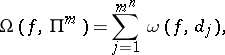whereis the oscillation of the functionon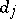(cf. Oscillation of a function). Then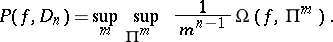If, then the functionis said to be of bounded (finite) Pierpont variation on, and the class of all such functions is denoted by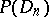. This definition was suggested by J. Pierpont . The classcontains as a subset the class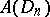of all functions of bounded Arzelà variation on.

How to Cite This Entry:
Pierpont variation. Encyclopedia of Mathematics. URL: http://encyclopediaofmath.org/index.php?title=Pierpont_variation&oldid=13447
This article was adapted from an original article by B.I. Golubov (originator), which appeared in Encyclopedia of Mathematics - ISBN 1402006098. See original article